# TSA Auto-Correlation Function VI

LabVIEW 2014 Advanced Signal Processing Toolkit Help

Edition Date: June 2014

Part Number: 372656C-01

»View Product InfoDownload Help (Windows Only)

Owning Palette: Correlation and Spectral Analysis VIs

Computes the normal or partial auto-correlation value of a univariate time series. Wire data to the Xt input to determine the polymorphic instance to use or manually select the instance.

Use the pull-down menu to select an instance of this VI.

 Select an instance TSA Auto-Correlation Function (Waveform)TSA Auto-Correlation Function (Array)

## TSA Auto-Correlation Function (Waveform)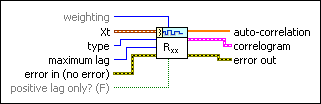weighting specifies to use the biased or unbiased weighting in the auto-correlation calculation. The default is Biased. Refer to the Details section for information about this parameter.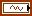Xt specifies the univariate time series.type specifies to compute the normal auto-correlation value or partial auto-correlation value of a time series. Refer to the Details section for information about how this VI computes the two types of auto-correlation values.maximum lag specifies the maximum lag to use in computing the auto-correlation value. The default is –1, which means the maximum lag equals N–1, where N is the length of the input time series.error in describes error conditions that occur before this node runs. This input provides standard error in functionality.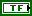positive lag only? specifies to return the auto-correlation with lag being positive or with lag being positive and negative. The default is FALSE. This option is available only when type is Normal.auto-correlation returns the auto-correlation value of the input time series.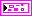correlogram returns, on an XY graph, the auto-correlation values against the lag.error out contains error information. This output provides standard error out functionality.

## TSA Auto-Correlation Function (Array)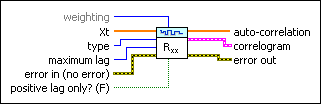weighting specifies to use the biased or unbiased weighting in the auto-correlation calculation. The default is Biased. Refer to the Details section for information about this parameter.Xt specifies the univariate time series.type specifies to compute the normal auto-correlation value or partial auto-correlation value of a time series. Refer to the Details section for information about how this VI computes the two types of auto-correlation values.maximum lag specifies the maximum lag to use in computing the auto-correlation value. The default is –1, which means the maximum lag equals N–1, where N is the length of the input time series.error in describes error conditions that occur before this node runs. This input provides standard error in functionality.positive lag only? specifies to return the auto-correlation with lag being positive or with lag being positive and negative. The default is FALSE. This option is available only when type is Normal.auto-correlation returns the auto-correlation value of the input time series.correlogram returns, on an XY graph, the auto-correlation values against the lag.error out contains error information. This output provides standard error out functionality.

## TSA Auto-Correlation Function Details

This VI computes the normal auto-correlation value of a univariate time series according to the following equation: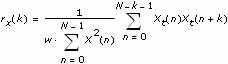where N is the number of samples of the input time series Xt. w is the weight. w=1 when weighting is biased.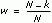when weighting is unbiased.

You can consider the partial auto-correlation rkk (k=1, 2, …) at lag k of a univariate time series as the correlation between Xt and qkXt with the correlation between the observations qXt, q2Xt, …, qk–1Xt removed. q is the delay operator.

This VI computes the partial auto-correlation value of a univariate time series according to the following equation: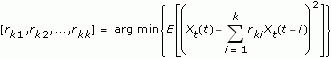where Xt is the univariate time series. E[X] denotes the expectation value of X.

## Example

Refer to the Correlation Analysis VI in the labview\examples\Time Series Analysis\TSAGettingStarted directory for an example of using the TSA Auto-Correlation Function VI.